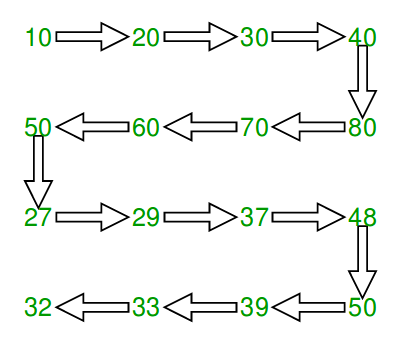# Print matrix in snake pattern

• Difficulty Level : Easy
• Last Updated : 29 Apr, 2021

Given an n x n matrix .In the given matrix, you have to print the elements of the matrix in the snake pattern.

Examples :

Attention reader! Don’t stop learning now. Get hold of all the important DSA concepts with the DSA Self Paced Course at a student-friendly price and become industry ready.  To complete your preparation from learning a language to DS Algo and many more,  please refer Complete Interview Preparation Course.

In case you wish to attend live classes with experts, please refer DSA Live Classes for Working Professionals and Competitive Programming Live for Students.

```Input :mat[][] = { {10, 20, 30, 40},
{15, 25, 35, 45},
{27, 29, 37, 48},
{32, 33, 39, 50}};

Output : 10 20 30 40 45 35 25 15 27 29
37 48 50 39 33 32

Input :mat[][] = { {1, 2, 3},
{4, 5, 6},
{7, 8, 9}};
Output : 1 2 3 6 5 4 7 8 9```We traverse all rows. For every row, we check if it is even or odd. If even, we print from left to right else print from right to left.

## C++

 `// C++ program to print matrix in snake order``#include ``#define M 4``#define N 4``using` `namespace` `std;`` ` `void` `print(``int` `mat[M][N])``{``    ``// Traverse through all rows``    ``for` `(``int` `i = 0; i < M; i++) {`` ` `        ``// If current row is even, print from``        ``// left to right``        ``if` `(i % 2 == 0) {``            ``for` `(``int` `j = 0; j < N; j++)``                ``cout << mat[i][j] << ``" "``;`` ` `        ``// If current row is odd, print from``        ``// right to left``        ``} ``else` `{``            ``for` `(``int` `j = N - 1; j >= 0; j--)``                ``cout << mat[i][j] << ``" "``;``        ``}``    ``}``}`` ` `// Driver code``int` `main()``{``    ``int` `mat[][] = { { 10, 20, 30, 40 },``                    ``{ 15, 25, 35, 45 },``                    ``{ 27, 29, 37, 48 },``                    ``{ 32, 33, 39, 50 } };`` ` `    ``print(mat);``    ``return` `0;``}`

## Java

 `// Java program to print matrix in snake order``import` `java.util.*;``class` `GFG``{``    ``static` `void` `print(``int` `[][] mat)``    ``{``        ``// Traverse through all rows``        ``for` `(``int` `i = ``0``; i < mat.length; i++)``        ``{`` ` `            ``// If current row is even, print from``            ``// left to right``            ``if` `(i % ``2` `== ``0``)``            ``{``                ``for` `(``int` `j = ``0``; j < mat[``0``].length; j++)``                    ``System.out.print(mat[i][j] +``" "``);`` ` ` ` `            ``// If current row is odd, print from``            ``// right to left``            ``}``            ``else``            ``{``                ``for` `(``int` `j = mat[``0``].length - ``1``; j >= ``0``; j--)``                    ``System.out.print(mat[i][j] +``" "``);``            ``}``        ``}``    ``}`` ` `    ``// Driver code``    ``public` `static` `void` `main(String[] args)``    ``{``        ``int` `mat[][] = ``new` `int``[][]``        ``{``            ``{ ``10``, ``20``, ``30``, ``40` `},``            ``{ ``15``, ``25``, ``35``, ``45` `},``            ``{ ``27``, ``29``, ``37``, ``48` `},``            ``{ ``32``, ``33``, ``39``, ``50` `}``        ``};`` ` `        ``print(mat);``    ``}``}``/* This code is contributed by Mr. Somesh Awasthi */`

## Python3

 `# Python 3 program to print``# matrix in snake order``M ``=` `4``N ``=` `4`` ` `def` `printf(mat):``    ``global` `M, N``     ` `    ``# Traverse through all rows``    ``for` `i ``in` `range``(M):``         ` `        ``# If current row is``        ``# even, print from``        ``# left to right``        ``if` `i ``%` `2` `=``=` `0``:``            ``for` `j ``in` `range``(N):``                ``print``(``str``(mat[i][j]),``                          ``end ``=` `" "``)`` ` `        ``# If current row is ``        ``# odd, print from``        ``# right to left``        ``else``:``            ``for` `j ``in` `range``(N ``-` `1``, ``-``1``, ``-``1``):``                ``print``(``str``(mat[i][j]), ``                          ``end ``=` `" "``)`` ` `# Driver code``mat ``=` `[[ ``10``, ``20``, ``30``, ``40` `],``       ``[ ``15``, ``25``, ``35``, ``45` `],``       ``[ ``27``, ``29``, ``37``, ``48` `],``       ``[ ``32``, ``33``, ``39``, ``50` `]]`` ` `printf(mat)`` ` `# This code is contributed``# by ChitraNayal`

## C#

 `// C# program to print ``// matrix in snake order``using` `System;``class` `GFG``{``    ``static` `void` `print(``int` `[,]mat)``    ``{``        ``// Traverse through all rows``        ``for` `(``int` `i = 0; ``                 ``i < mat.GetLength(0); i++)``        ``{`` ` `            ``// If current row is ``            ``// even, print from ``            ``// left to right``            ``if` `(i % 2 == 0)``            ``{``                ``for` `(``int` `j = 0; ``                         ``j < mat.GetLength(1); j++)``                    ``Console.Write(mat[i, j] + ``" "``);`` ` ` ` `            ``// If current row is ``            ``// odd, print from ``            ``// right to left``            ``}``            ``else``            ``{``                ``for` `(``int` `j = mat.GetLength(1) - 1; ``                         ``j >= 0; j--)``                    ``Console.Write(mat[i, j] + ``" "``);``            ``}``        ``}``    ``}`` ` `    ``// Driver code``    ``public` `static` `void` `Main()``    ``{``        ``int` `[,]mat = {{ 10, 20, 30, 40 },``                      ``{ 15, 25, 35, 45 },``                      ``{ 27, 29, 37, 48 },``                      ``{ 32, 33, 39, 50 }};`` ` `        ``print(mat);``    ``}``}`` ` `// This code is contributed``// by ChitraNayal`

## PHP

 `= 0; ``\$j``--)``                ``echo` `\$mat``[``\$i``][``\$j``] , ``" "``;``        ``}``    ``}``}`` ` `// Driver code``\$mat` `= ``array``(``array``(10, 20, 30, 40),``             ``array``(15, 25, 35, 45),``             ``array``(27, 29, 37, 48),``             ``array``(32, 33, 39, 50));`` ` `printLN(``\$mat``);`` ` `// This code is contributed by ajit ``?>`

## Javascript

 ``

Output :

`10 20 30 40 45 35 25 15 27 29 37 48 50 39 33 32 `

This article is contributed by Rakesh Kumar. If you like GeeksforGeeks and would like to contribute, you can also write an article using write.geeksforgeeks.org or mail your article to contribute@geeksforgeeks.org. See your article appearing on the GeeksforGeeks main page and help other Geeks.